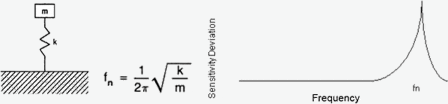Home/Resources/Ask The Experts/Figure of Merit for a piezoelectric vibration transducer (accelerometer)

# Figure of Merit for a piezoelectric vibration transducer (accelerometer)

### Question

What is the background on the term "Figure of Merit." I have seen this term in industry publications without any real explanation. Can you provide a brief explanation on this?

Figure of merit is a function of the sensitivity times the resonant frequency squared (Sq x fn2) where Sq is the charge sensitivity of a piezoelectric accelerometer and fn is the mounted resonant frequency of a piezoelectric accelerometer. Figure of merit is a measure of efficiency of a design.

The higher the figure of merit for a piezoelectric vibration transducer, the more efficient is the design which results in a greater frequency range for equivalent sensitivities. Higher figure of merit designs also provide for significantly reduced size and weight for equivalent sensitivities and frequency response.A piezoelectric accelerometer is an undamped spring mass system where its seismic mass "m" and mechanical stiffness "k" is also tied to figure of merit.As the seismic mass "m" decreases, the mounted resonant frequency "fn" increases. As the mechanical stiffness "k" increases, the mounted resonant frequency "fn" increases.

For a piezoelectric accelerometer, the charge sensitivity is proportional to the seismic mass "m" and the type of piezoelectric sensing element utilized. Increased seismic mass "m" results in higher charge sensitivity for a given piezoelectric sensing element.

As seen above, the frequency response is inversely proportional to the seismic mass "m" and proportional to the mechanical stiffness "k."

Summary:
The thing to remember about a piezoelectric vibration transducer (accelerometer) is that a more efficient piezoelectric sensing element will require less seismic mass for a given charge sensitivity. Reduced seismic mass leads to a higher mounted resonant frequency (more working bandwidth) along with smaller device size and device weight. PZT based ferroelectric ceramics are considered the best material to use, to achieve the highest charge coefficient, up to 500°F (260°C).